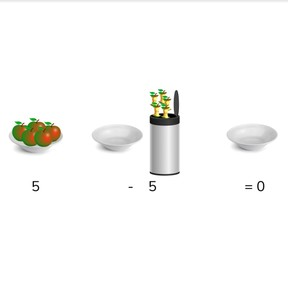Subtraction to 10 with result of 0 or 1

# Subtraction to 10 with result of 0 or 18,000 schools use Gynzy92,000 teachers use Gynzy1,600,000 students use Gynzy

## General

Students learn to subtract numbers to 10 whose difference is 0 or 1.

1.OA.A
1.OA.C.5

## Introduction

Students stand (could be in a circle). Take a ball and throw it to a student saying "one". The student who catches the ball throws it to another student, saying "two". Continue counting forward to 10. When 10 is reached, the student throwing must start counting down, back to 0.

## Instruction

Explain to students that when you subtract a number equal to the quantity you have, you have 0 left. Show this with the example of the dogs and the doghouse. Explain that this is subtracting to 0. Then discuss the image of bananas with the students. Ask how many bananas need to be taken away to subtract to 0. Next show a subtraction problem to 0 without visual support. Explain that when the number after the minus sign is the same as the number before the minus sign, that the answer is always zero.

Next, explain to students that when the number you take away is 1 less than the number you have, you have 1 left. Show this with the example of the dogs and the doghouse. Explain that this is subtraction to 1. Show the example of bananas and ask how many they need to take away to subtract to 1. Next, show a subtraction problem to 1 without visual support. Explain that when the number after the minus sign is 1 less than the number before the minus sign, that the answer is always 1.
Students must next determine which subtraction problems are subtraction to 0, and which are subtraction to 1. Drag the subtraction problems to the correct column. Finally, do a selection of subtraction problems with the class, and make sure students understand how to determine if the subtraction problem is to 0 or 1.

To check that students understand subtraction with numbers to 10 with a result of 0 or 1 you can ask the following questions:
- What is subtraction to 0?
- What is subtraction to 1?
- Is 8-7 subtraction to 0 or 1? How do you know?

## Guided practice

Students first practice with subtraction to 0 and then with subtraction to 1. In the third exercise, they are given subtraction problems both to 0 and 1.

## Closing

Discuss with students why it is important to be able to subtract numbers to 10 with a difference of 0 or 1. Students must then play a game, in which they determine if a subtraction problem is to 0 or 1. If the subtraction problem is to 0, they must sit down. If the subtraction problem is to 1, they must stand up. You can ask students how they know if it is subtraction to 0 or 1.

## Teaching tips

Students who have difficulty with this subtraction goal can be given blocks or other manipulatives. They can then visually see how many are left (0 or 1) when they take the second number away from the first.

## Instruction materials

Optional: blocks or manipulatives

### The online teaching platform for interactive whiteboards and displays in schools

• Save time building lessons

• Manage the classroom more efficiently

• Increase student engagement